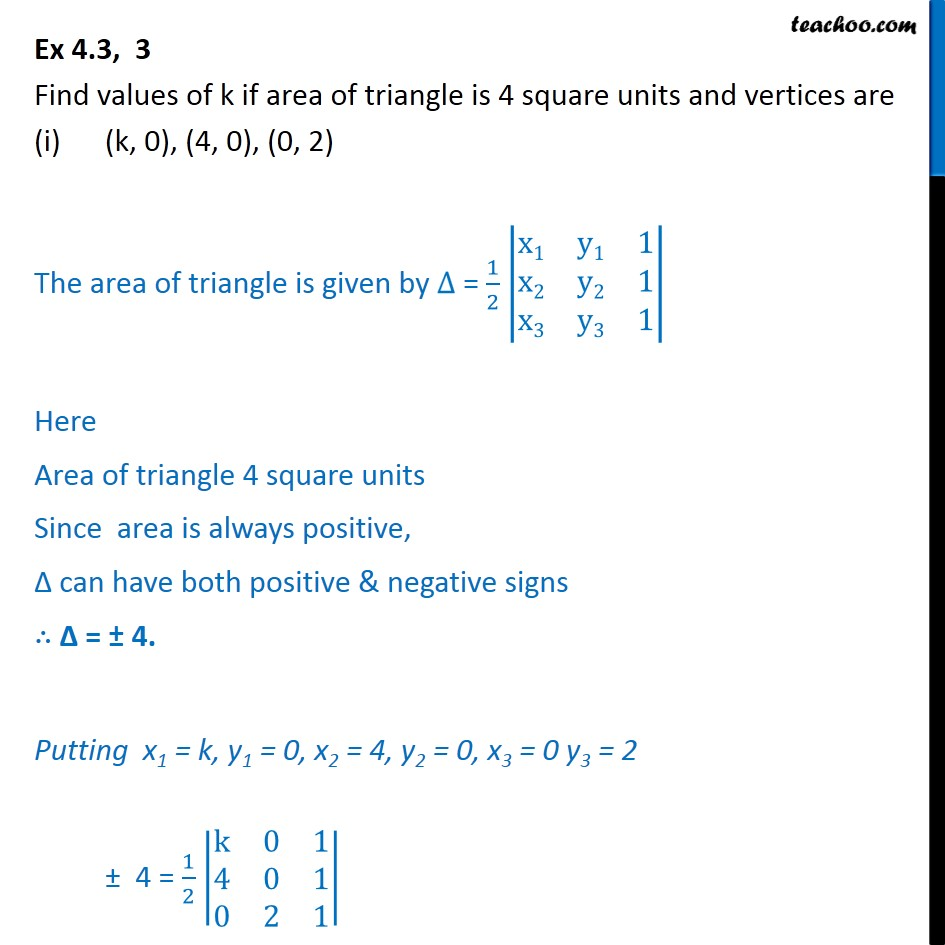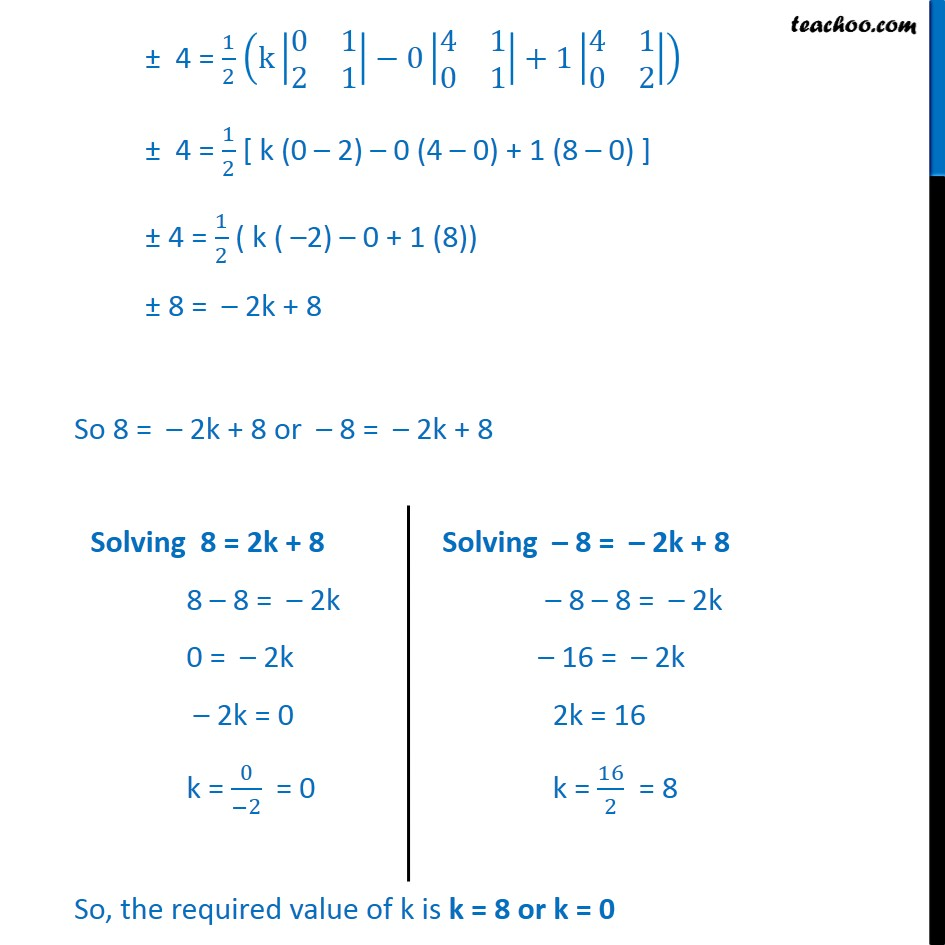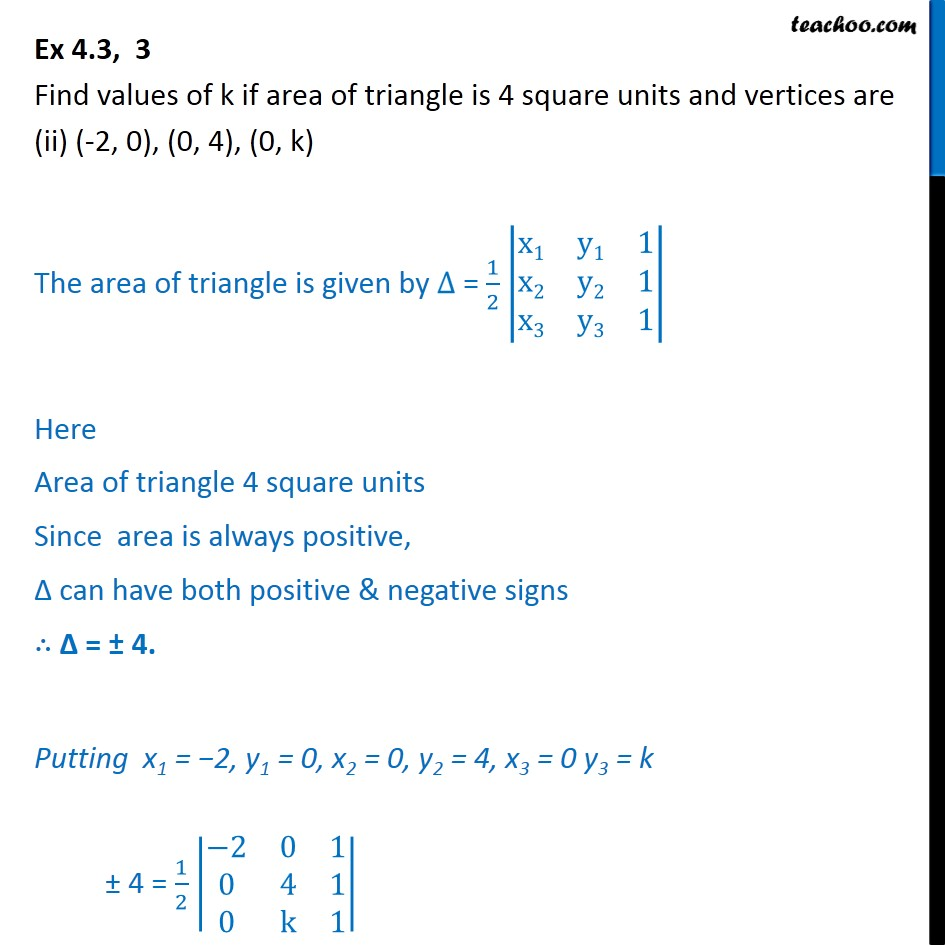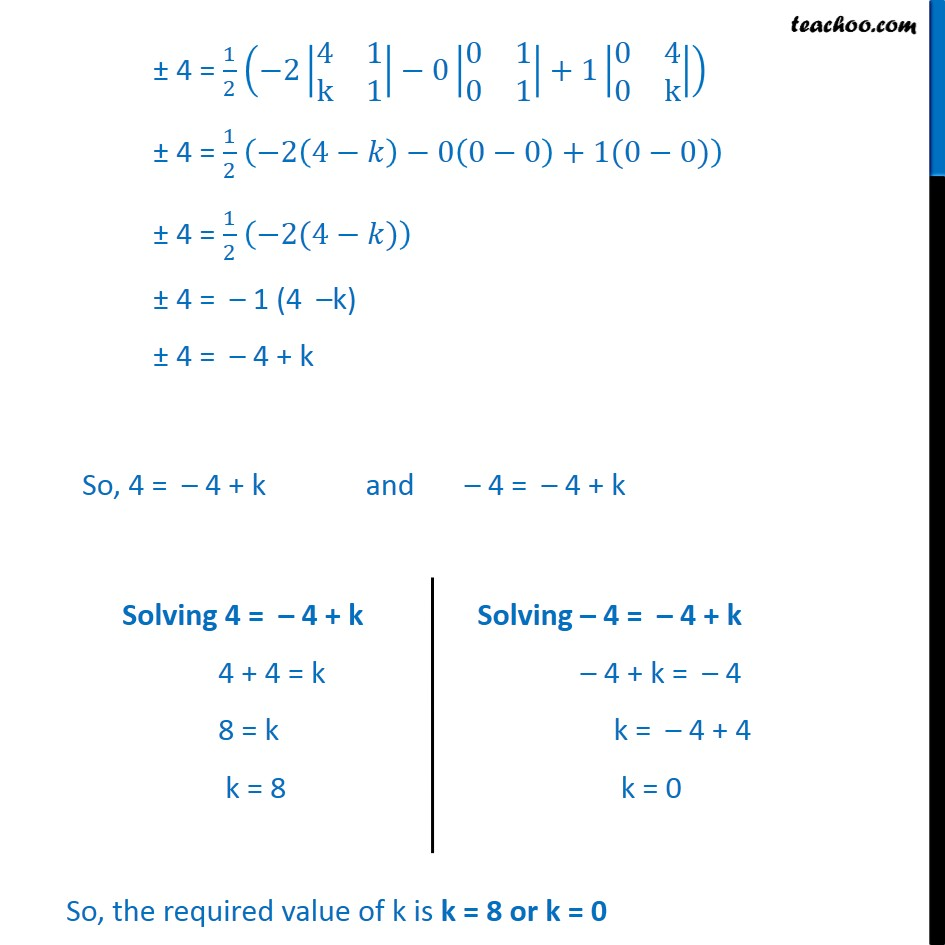1. Class 12
2. Important Question for exams Class 12
3. Chapter 4 Class 12 Determinants

Transcript

Ex 4.3, 3 Find values of k if area of triangle is 4 square units and vertices are • (k, 0), (4, 0), (0, 2) The area of triangle is given by ∆ = 1﷮2﷯ x1﷮y1﷮1﷮x2﷮y2﷮1﷮x3﷮y3﷮1﷯﷯ Here Area of triangle 4 square units Since area is always positive, ∆ can have both positive & negative signs ∴ Δ = ± 4. Putting x1 = k, y1 = 0, x2 = 4, y2 = 0, x3 = 0 y3 = 2 ± 4 = 1﷮2﷯ k﷮0﷮1﷮4﷮0﷮1﷮0﷮2﷮1﷯﷯ ± 4 = 1﷮2﷯ k 0﷮1﷮2﷮1﷯﷯−0 4﷮1﷮0﷮1﷯﷯+1 4﷮1﷮0﷮2﷯﷯﷯ ± 4 = 1﷮2﷯ [ k (0 – 2) – 0 (4 – 0) + 1 (8 – 0) ] ± 4 = 1﷮2﷯ ( k ( –2) – 0 + 1 (8)) ± 8 = – 2k + 8 So 8 = – 2k + 8 or – 8 = – 2k + 8 Ex 4.3, 3 Find values of k if area of triangle is 4 square units and vertices are (ii) (-2, 0), (0, 4), (0, k) The area of triangle is given by ∆ = 1﷮2﷯ x1﷮y1﷮1﷮x2﷮y2﷮1﷮x3﷮y3﷮1﷯﷯ Here Area of triangle 4 square units Since area is always positive, ∆ can have both positive & negative signs ∴ Δ = ± 4. Putting x1 = −2, y1 = 0, x2 = 0, y2 = 4, x3 = 0 y3 = k ± 4 = 1﷮2﷯ −2﷮0﷮1﷮0﷮4﷮1﷮0﷮k﷮1﷯﷯ ± 4 = 1﷮2﷯ −2 4﷮1﷮k﷮1﷯﷯−0 0﷮1﷮0﷮1﷯﷯+1 0﷮4﷮0﷮k﷯﷯﷯ ± 4 = 1﷮2﷯ −2 4−𝑘﷯−0 0−0﷯+1(0−0)﷯ ± 4 = 1﷮2﷯ −2(4−𝑘)﷯ ± 4 = – 1 (4 –k) ± 4 = – 4 + k So, 4 = – 4 + k and – 4 = – 4 + k

Chapter 4 Class 12 Determinants

Class 12
Important Question for exams Class 12

About the AuthorDavneet Singh
Davneet Singh is a graduate from Indian Institute of Technology, Kanpur. He has been teaching from the past 9 years. He provides courses for Maths and Science at Teachoo.